# Hurwitz equation

(diff) ← Older revision | Latest revision (diff) | Newer revision → (diff)

Markoff–Hurwitz equation, Markov–Hurwitz equation

A Diophantine equation (cf. Diophantine equations) of the form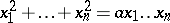(a1)

for fixed,. The casewas studied by A.A. Markoff [A.A. Markov] [a1] because of its relation to Diophantine approximations (cf. also Markov spectrum problem). More generally, these equations were studied by A. Hurwitz [a2]. These equations are of interest because the set of integer solutions to (a1) is closed under the action of the group of automorphismsgenerated by the permutations of the variables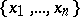, sign changes of pairs of variables, and the mapping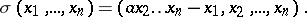If (a1) has an integer solutionandis not the trivial solution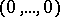, then its-orbit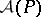is infinite. Hurwitz showed that if (a1) has a non-trivial integer solution, then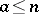; and if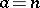, then the full set of integer solutions is the-orbit of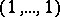together with the trivial solution. N.P. Herzberg [a3] gave an efficient algorithm to find pairs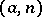for which the Hurwitz equation has a non-trivial solution. Hurwitz also showed that for any pairthere exists a finite set of fundamental solutions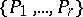such that the orbitsare distinct and the set of non-trivial integer solutions is exactly the union of these orbits. A. Baragar [a4] showed that for anythere exists a pairsuch that (a1) has at leastfundamental solutions.

D. Zagier [a5] investigated the asymptotic growth for the number of solutions to the Markov equation (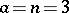) below a given bound, and Baragar [a6] investigated the cases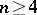.

There are a few variations to the Hurwitz equations which admit a similar group of automorphisms. These include variations studied by L.J. Mordell [a7] and G. Rosenberger [a8]. L. Wang [a9] studied a class of smooth variations.